Chi Square/ Cramer’s V/ PSI

Example Codes:

# Association Between Categorical Variables

Existence of association between two categorical variables implies that the change of level of one variable is related to the change of value of another variable. Tests or measurements of association reveals the relationship between two variables of interest, for example, whether smoking status effects the occurrence of a certain disease, whether gender is related to obesity, and whether an exposure is associated with a health outcome.

Determining the frequencies of data values and building a frequency table gives a preliminary overview of the relationship between two variables. If there is no association, the distribution of the first variable is the same regardless of the level of the other variable.

# Chi Square Test

Chi-squared test is a statistical hypothesis test for the association between two categorical variables. The null hypothesis of Chi-squared test is that there is no association between the two variables, whereas the alternative hypothesis is that the association exists.

Suppose a researcher is investigating the relationship between gender and survival of passengers in the Titanic crash, through Chi-squared test. The null hypothesis of the test is that gender and survival are not associated, which means that the probability of surviving the crash was the same across gender. The alternative hypothesis is that gender and survival are associated, which means the probability of surviving the Titanic crash was not the same for males and females.

The test statistics is: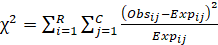,  following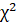distribution with degrees of freedom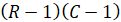.

Where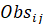is the observed frequency,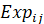is the expected count,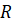is the number of rows of the frequency table and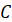is the number of columns.

If the corresponding p-value of the test statistic is less than the chosen significance level, then the association between the two variables is statistically significant.

# Measures of Association

## Cramer’s V

Cramer’s V is a measure of association between two nominal variables. For a frequency table with more than 2 rows and 2 columns, Cramer’s V is always non-negative and between 0 and 1. The value of Cramer’s V indicate the how strongly the association between the two categorical variables is.

Cramer’s V is computed as: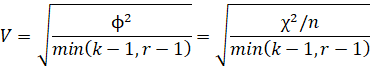, where:

-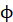is the PHI coefficient

-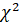is the test statistic of Chi-squared test

-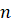is the number of observations

-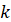is the number of columns

-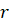is the number of rows

## PHI Coefficient

The PHI coefficient measures the association between two binary variables.  For a 2*2 frequency table, the value of Pearson correlation coefficientis the same as the value of PHI coefficient.

Suppose we have a following frequency table:

 Y = 0 Y = 1 X = 0 a b X = 1 c d

, where a, b, c, d are the frequencies in corresponding groups.

Then the PHI coefficient is computed as:# Example Code in SAS

DATA PERSONS ; INPUT GROUP \$ SUCCESS \$ @@;

DATALINES ;

DRUG NO DRUG NO DRUG NO DRUG YES

DRUG YES DRUG YES DRUG YES DRUG YES

DRUG YES DRUG YES

PLACEBO NO PLACEBO NO PLACEBO YES PLACEBO YES

PLACEBO YES PLACEBO YES PLACEBO YES PLACEBO YES

PLACEBO YES PLACEBO YES

RUN ;

PROC FREQ DATA = PERSONS ;

TABLES GROUP * SUCCESS/ NOPERCENT NOCOL NOROW

CHISQ EXPECTED ;

RUN ;

# Example Code in R

install.packages("confintr")

install.packages("psych")

# Load package for function "cramersv"

library(confintr)

# Load package for function "phi"

library(psych)

# input data

success <- c("No", "No", "No", "Yes", "Yes", "Yes", "Yes", "Yes", "Yes", "Yes",

"No", "No", "Yes", "Yes", "Yes", "Yes", "Yes", "Yes", "Yes", "Yes")

group <- c("drug", "drug", "drug", "drug", "drug", "drug", "drug", "drug",

"drug", "drug", "placebo", "placebo", "placebo", "placebo",

"placebo", "placebo", "placebo", "placebo", "placebo", "placebo")

# create a dataframe

df <- data.frame(success, group)

# compute the contingency table

tbl <- table(df)

# calculate Cramer's V

cramersv(df)

# calculate PHI

phi(tbl)

# Reference

1.     Cramér, Harald. 1946. Mathematical Methods of Statistics. Princeton: Princeton University Press, page 282 (Chapter 21. The two-dimensional case).

2.     Yule, G. U. (1912). On the Methods of Measuring Association Between Two Attributes. Journal of the Royal Statistical Society, 75(6), 579. https://doi.org/10.2307/2340126